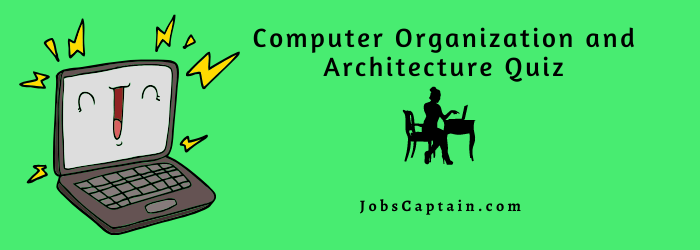# Computer Organization and Architecture QuizComputer Organization and Architecture Multiple Choice Questions and Answers pdf.  It contains well thought and well-explained computer science and programming objective quizzes.

## Computer Organization & Architecture Quiz with Answers

Let’s start Computer Organization and Architecture Quiz.

Question 1: How many digits are used in Binary number system?

(A) zero

(B) one

(C) two

(D) three

(C) two

Question 2: What type of computer uses the binary number system?

(A) Analog

(B) Super

(C) Intra

(D) Digital

(D) Digital

Question 3: What digit is called a bit?

(A) decimal

(B) binary

(C) octal

(B) binary

Question 4: Which component of the computer consists of physical entity of the device?

(A) software

(B) middleware

(C) hardware

(D) firmware

(C) hardware

Question 5: Which consists of instructions and data that computer manipulates to perform data processing tasks?

(A) software

(B) middleware

(C) hardware

(D) firmware

(A) software

Question 6: What is the term used for “sequence of instructions for the computer”?

(A) hardware

(B) program

(C) data

(D) instruction

(B) program

Question 7: What is concerned with the way the hardware components operate to form computer system?

(A) Computer organization

(B) Computer design

(C) Computer architecture

(D) Computer implementation

(A) Computer organization

Question 8: The manipulation of binary information is done by logic circuits called __________.

(A) blocks

(B) gates

(C) symbols

(D) functions

(B) gates

Question 9: Each gate can be represented in tabular form by __________.

(A) symbols

(B) function

(C) truth table

(D) logic

(C) truth table

Question 10: If both inputs A and B are equal to 1 in AND gate, the output is ___________.

(A) zero

(B) one

(C) don’t care

(D) binary

(B) one

Question 11: A Boolean function can be translated from ____________ expression into a logic diagram.

(A) boolean

(B) logical

(C) relational

(D) algebric

(D) algebric

Question 12: The map simplification method is also known as ___________ map.

(A) karnaugh

(B) algebric

(C) basic

(D) binary

(A) karnaugh

Question 13: The condition when it does not matter if the function produces 0 or 1 for a given minterm is?

(A) SOP

(B) POS

(C) NOR

(D) don’t care

(D) don’t care

Question 14: A _________ circuit is a connected arrangement of logic gates with a set of inputs and outputs.

(A) arithmetic

(B) logic

(C) combinational

(D) shift

(C) combinational

Question 15: The addition of 2 binary digits is done by ___________ circuit.

Question 16: A _________ is a combinational circuit that forms sum of 3 input bits.

Question 17: The storage element employed in clocked sequential circuit is called ___________.

(A) memory

(B) flipflop

(C) circuit

(D) subtractor

(B) flipflop

Question 18: Minterm is also called ________.

(A) standard product

(B) standard sum

(C) union

(D) difference

(A) standard product

Question 19: In flip-flop input R stands for __________.

(B) reset

(C) reimburse

(D) rectangle

(B) reset

Question 20: In T flip-flop T stands for?

(A) technical

(B) toggle

(C) trigger

(D) type edged

(B) toggle

Question 21: Special input terminal for setting the flip-flop is called _____________.

(A) clear

(B) set

(C) preset

(D) reset

(C) preset

Question 22: A _____________ circuit is an interconnection of flip-flops and gates.

(A) combinational

(B) arithmetic

(C) shift

(D) sequential

(D) sequential

Question 23: The state table of sequential circuit consists of __________ sections.

(A) one

(B) two

(C) three

(D) four

(D) four

Question 24: The ___________ state shows the states of flip-flops A and B at any given time t.

(A) input

(B) next

(C) present

(D) output

(C) present

Question 25: Short form of “American Standard Code for Information Interchange”.

(A) ASCFII

(B) ASCII

(C) ASCFI

(D) ASCI

(B) ASCII

Question 26: The state of the flip flop after the occurrence of a clock pulse is called __________ state .

(A) high level

(B) present

(C) next

(D) low level

(C) next

Question 27: Maxterm is also called ________.

(A) standard product

(B) standard sum

(C) union

(D) differnce

(B) standard sum

Question 28: The multiplexer is also called ________ selector since it selects one of many data inputs.

(A) line

(B) data

(C) binary

(D) octal

(B) data

Question 29: A _______ is a digital circuit that performs the inverse operation of a decoder.

(A) multiplexer

(C) subtractor

(D) encoder

(D) encoder

Question 30: A ________ is a group of flip-flops.

(A) memory

(B) circuit

(C) register

(D) bits